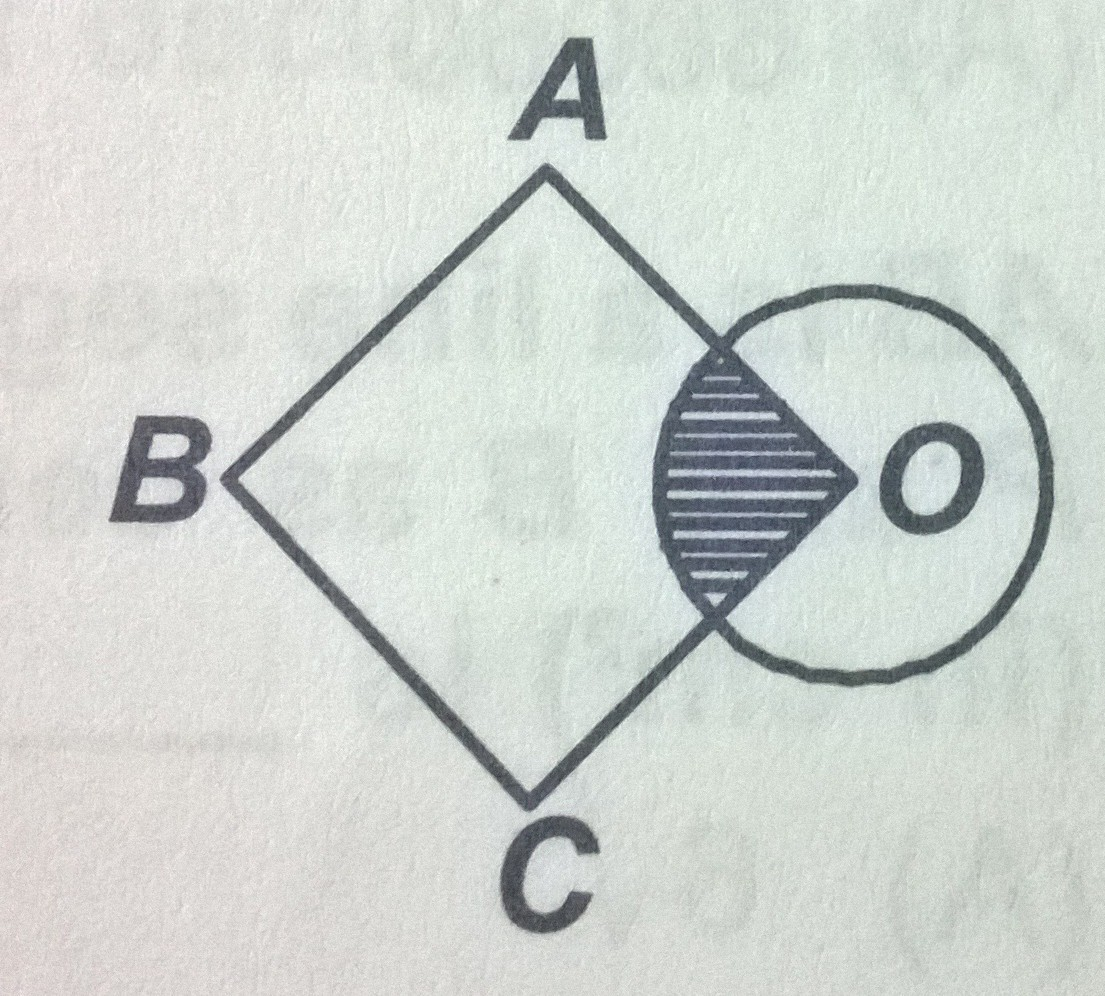# Sector

Geometry Level 2O is the centre of a circle of diameter 4 units and OABC is a square, if the shaded area is $\frac{1}{3}$ area of the square, then the side of the square is in the form $\sqrt[x]{ y \pi }$

Find $x + y$

×